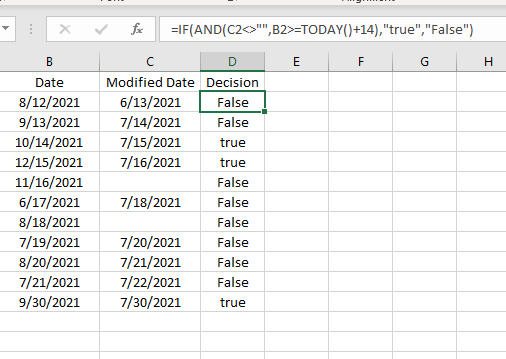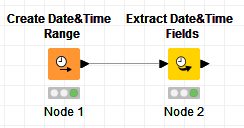# Formula write with Today() and AND

Hi,
How can I write multiple condition formula ?

=IF(AND(C2<>"",B2>=TODAY()+14),“true”,“False”)

And is there any built in function in KNIME to find out WEEKDAY (Number) from Today date?It depends which node you are using. In the Column Expressions for example, you can use the javascript format of:

``````if(conditions) {
This part will execute if conditions are true
} else {
This part will execute if conditions are not true
}
``````

You can also have different conditions with different results:

``````if(conditions 1) {
This part will execute if conditions 1 are true
} else if (conditions 2) {
This part will execute if conditions 2 are true
} else {
This part will execute if none of the above sets of conditions are not true
}
``````

You can do this in 2 steps.
Get the current date first via the Create Date&Time Range:

You just need to make sure you configure these 4 highlighted items.

Then use the Extract Date&Time Fields, and you can extract the Week (or other fields):

A simple test would look like this:And results would be:4 Likes

thank you @bruno29a for your suggestion. I have an issue with java script. When I am writing the below script, it is showing error message

if ((column(“Modified Date”)<> " " And column(“Date”)>= today()+14)) { “True” } else { “False”}

Error Message:

Errors in code. Please fix the expression.
Expected an operand’ but found '>
’ if ((column(“Modified Date”)<> " " And column(“Date”)>= today()+14)) {“True”} else {“False”}
^ at line 1 at column 30

1 Like

The problem is solved.

if (and(not(column(“Modified Date”))== “”,column(“Date”)>= column(“two weeks from today”))) {“True”} else {“False”}

2 Likes

This topic was automatically closed 7 days after the last reply. New replies are no longer allowed.